# Python Program to Create Pyramid Patterns

In this tutorial you will learn about the Python Program to Create Pyramid Patterns and its application with practical example.

In this tutorial, we will learn to create a Python Program to Create full Pyramid Patterns  using python Programming.

## Prerequisites

Before starting with this tutorial we assume that you are best aware of the following Python programming topics:

• Python Operator.
• Basic Input and Output
• Basic Python  programming.
• Python if-else statements.
• Python Loop.
• List in Python.

## What is Pyramid?

Pyramid is a polygon structure and formed by connecting a polygonal base and a point. Each base edge and apex form a triangle.To print pyramid patterns in Python we  will use  two nested for loops and a while loop.The outermost loop is responsible for rows of pyramid triangle where inner one  will print the required number of stars (*) in each row.

## Python Program to Create Pyramid Patterns

In this tutorial we will create a program of full Pyramid using nested for loop and a while loop. We would first declared and initialized the required variables. Then we will create the Pyramid using below program.Let’s have a look at the code.

## Output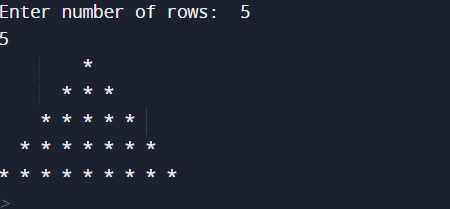In our program we are printing Full Pyramid using nested for and while loop. We would first declared and initialized the required variables. First of all we take value form user to print number of rows and later we will print a Full Pyramid.

First, we get the  number of rows of the pyramid  from the user.As shown in image below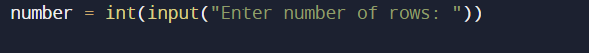The first  for loop starts from i = 1 to i = number+ 1.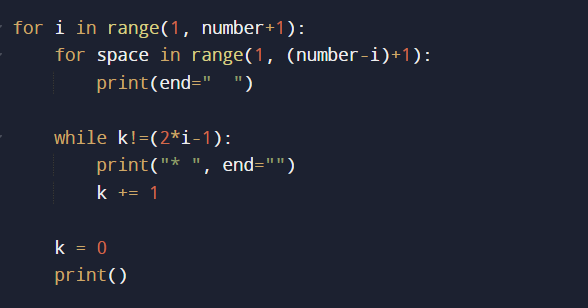the inner for loops, prints the spaces for each row using formula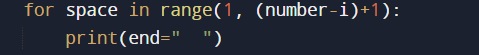(number-i)+1, here number is the total number of rows and i is the current row number.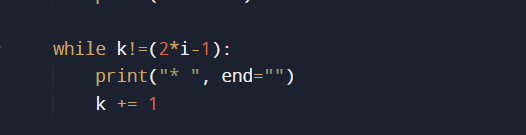The while loop prints number of stars using  “2 * i – 1″. This formula gives the number of stars for each row.And the final output of our program is like that.

In this tutorial we have learn about the Python Program to Create Pyramid Patterns and its application with practical example. I hope you will like this tutorial.